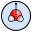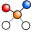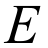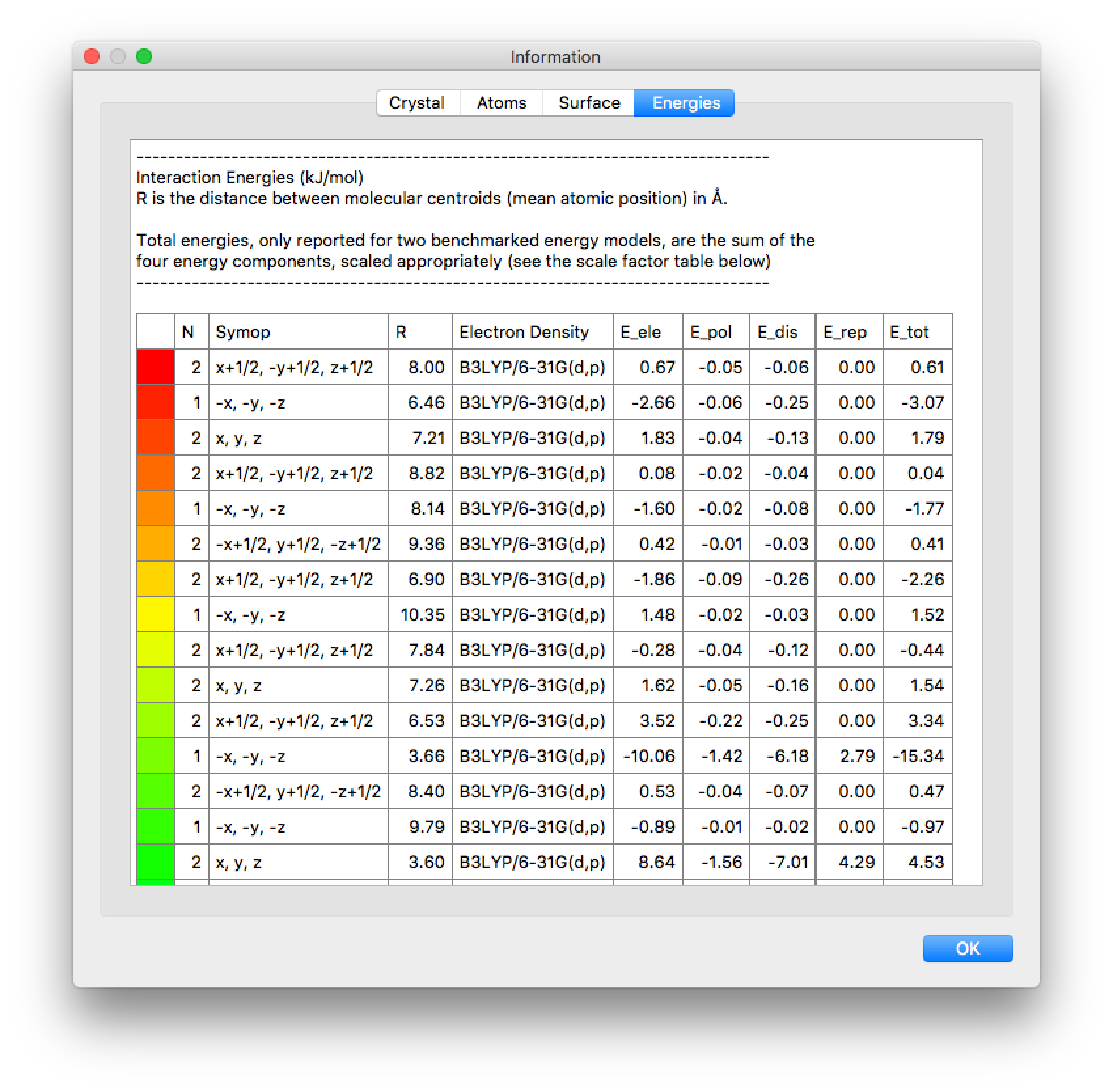# Lattice Energies

Lattice energies of molecular crystals can also be obtained with CrystalExplorer17, using the same CE-B3LYP interaction energies that underpin the calculation of energy frameworks.

Using four different benchmark sets of molecular crystals, we have established the level of confidence for lattice energies estimated using CE-B3LYP model energies and experimental crystal structures. They compare very well with available benchmark estimates derived from sublimation enthalpies, and in many cases they are comparable with, and sometimes better than, more computationally demanding approaches, such as those based on periodic DFT+dispersion methodologies. The performance over the complete set of 110 crystals indicates a mean absolute deviation from benchmark energies of only 6.6 kJ/mol. That reference also discussed applications to polymorphic crystals and some much larger molecules.

At present the calculation of lattice energies has not been automated, but it is a relatively straightforward procedure. CE-B3LYP lattice energies are computed by direct summation of interaction energies in CrystalExplorer over molecules $\text{B}$ interacting with a central molecule $\text{A}$ until $E_{\text{latt}}$ is converged to better than ~1 kJ/mol, using a cutoff based on the separation of molecular centroids, $r_\text{AB}$. Sums over $E_\text{dis}$ and $E_\text{rep}$ converge rapidly, but the sum over $E_{\text{ele}}$ terms is much longer range, with highly dipolar compounds (e.g., HCN, formamide, zwitterionic amino acids) requiring much greater cutoff distances than nondipolar molecules (e.g., anthracene, coronene). For molecules separated by more than 12 Å, $E_\text{ele}$ is computed using a distributed multipole model of the electron density.

## Calculating Lattice Energies: Only one unique molecule in the unit cell - $Z' = 1$​

1. The first step is to construct a cluster of sufficient size around a molecule, typically with the Generate Atoms Within Radiusbutton, making sure that all molecule fragments are completed. For non-dipolar molecules a radius of 20 Å should be adequate, but more dipolar molecules (e.g., amino acids, zwitterions) may require a cluster radius of 30 Å or more.

2. With the central molecule still selected, clicking on Calculate Energieswill open the dialog box for energies. Select Accurate [B3LYP/6-31G(d,p)]. This is the only energy model that has been calibrated against experimental sublimation enthalpies. Clicking on OK will initiate wavefunction calculation, followed by computation of all unique pair energies of the central molecule with other molecules in the cluster. This (naturally) takes a little time, and progress of the computation can be followed by the dialog and progress bar at the bottom of the graphics window.1. The Information window will open automatically when all unique pair energies have been computed. An example for formamide is shown on the right. The text in this window can be copied and pasted into a spreadsheet application, and from this the lattice energy obtained as one-half the product of the two columns labelled N and Etot, where N is the number of molecule pairs in the cluster with that particular interaction energy. In the same manner the lattice energy can be broken down into separate contributions, electrostatic, dispersion, etc. (Note that the number of decimal places for energy quantities in the Information window can be modified using the Preferences dialog).

2. Pay careful attention to whether the lattice energy estimate you have obtained is adequately converged. You can do this by sorting the energies by separation of molecular pairs, and computing partial sums, or by extending the cluster by several Å, computing the additional energies and the revised estimate of the lattice energy, then repeating until convergence. Suitably converged lattice energies should show consistent changes of less than 1 kJ mol–1.

## Calculating Lattice Energies: More than one unique molecule in the unit cell - $Z' > 1$​

The procedure here is similar to that above, but more complicated because separate clusters need to be created for each unique molecule.

For each unique molecule you need to do the following:

1. Select the molecule and create a suitable cluster around it as above. Don't forget to Complete fragments.2. Assuming the central molecule is still selected, click on thebutton in the toolbar. This will now bring up a dialog asking if you wish to change the charge and/or multiplicity of any of the molecular species. For neutral closed shell species the answer should be No, but if you are working with a radical structure you will need to respond Yes and set the correct multiplicities here.
note

Lattice energies are not yet implemented for charged species such as salts, which present more sophisticated approaches to summing the electrostatic energy.

3. After clicking OK, the Calculate Interaction Energy dialog will appear as above. Choose the energy model, click OK, and wavefunctions will be computed for all unique molecules in the cluster. Interaction energies between the selected molecule and its neighbours will be computed, and energies will appear in the Information dialog, as above. This text should be copied and pasted into a spreadsheet application, and from this the lattice energy sum for this unique molecule is again obtained as one-half the product of the two columns labelled $N$ and $E_\text{tot}$.
4. Repeat the steps above for all other unique molecules, after which you should have obtained converged lattice energy sums for each unique molecule in the unit cell. Some care will now be needed to obtain the lattice energy for the crystal. For example, for two molecules of the same kind in the unit cell, the lattice energy will be the average of the lattice sums for the two individual molecules. For co-crystals, the stoichiometry determines how separate lattice sums are added to give the lattice energy for the crystal. (If this isn't clear, consider the relationship of the crystal lattice energy to the sublimation process, and ask yourself how many different molecules are obtained upon sublimation. As an example, a 1:1 co-crystal $\text{A}$:$\text{B}$ may have two different molecules of $\text{A}$ and two different molecules of $\text{B}$, in which case the lattice energy for the crystal will be the sum of the average lattice sums for each of molecules $\text{A}$ and $\text{B}$, reflecting the 1:1 stoichiometry).

## Polar space groups require special consideration​

For unit cells with nonzero dipole moments (i.e., polar space groups such as $\text{P2}_1$, $\text{Pna2}_1$, and $\text{Fdd2}$) the simple lattice summation described above is not representative of the entire crystal, and an additional consideration is required, the cell dipole energy, $E_\text{cell}$ dipole. Numerous examples of the additional term can be found in the Supporting Information to the lattice energy paper. Calculation of this term is straightforward, but it requires an estimate of the unit cell dipole moment. This can be obtained by summing the components along the polar direction of all molecular dipole moments in the unit cell, and that information is easily obtained from the Gaussian output files for each molecule.

## Relaxation energy and conformational energy differences​

The lattice energy can be related to the sublimation enthalpy by taking into account the electronic relaxation energy associated with the crystal to gas transition, as well the difference between vibrational energies for the two phases,

$\Delta H_{sub}(T) = −E_{lat} + \Delta E_{rel} + \Delta E_{vib} + 4RT$

and this can be (reasonably well) approximated by

$\Delta H_{sub}(T) = −E_{lat} + \Delta E_{rel} + 2RT$

The important point here is that for crystals where little molecular geometry change occurs between crystal and gas phase, the sublimation enthalpy is a positive quantity, whose magnitude at room temperature is ~5 kJ/mol less than that of the lattice energy.

Conformational polymorphism is common in pharmaceutical compounds, a consequence of their considerable internal flexibility, and any attempt to estimate relative polymorph stability must also incorporate the energy differences between the different conformations found in the polymorphs. We have discussed one way of estimating $\Delta E_{rel}$, with application to the ROY polymorphs.